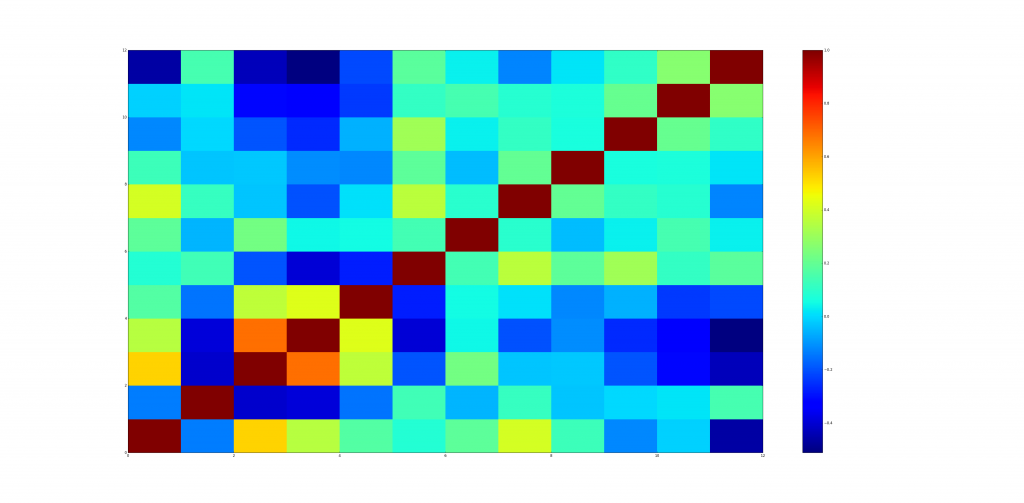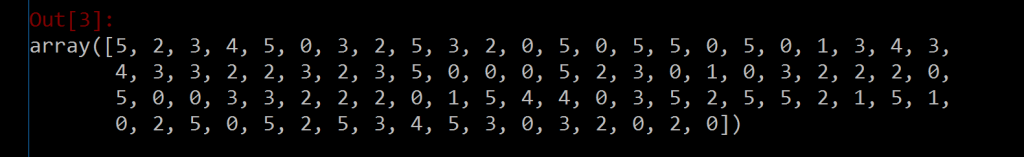# Project | Scikit-learn – Whisky Clustering

Introduction | Scikit-learn

Scikit-learn is a machine learning library for Python.It features various classification, regression and clustering algorithms including support vector machines, random forests, gradient boosting, k-means and DBSCAN, and is designed to interoperate with the Python numerical and scientific libraries NumPy and SciPy.Learn more on Scikit-learn from here.

Case Study | Clustering Whiskey

Aim & Description: Scotch whisky is prized for its complexity and variety of flavors.And the regions of Scotland where it is produced are believed to have distinct flavor profiles.In this case study, we will classify scotch whiskies based on their flavor characteristics.The dataset we’ll be using contains a selection of scotch whiskies from several distilleries, and we’ll attempt to cluster whiskies into groups that are similar in flavor.This case study will deepen your understanding of Pandas, NumPy, and scikit-learn, and perhaps of scotch whisky.

Source: Download the whiskey regions dataset and whiskey varieties dataset.We will put these datasets in the working path directory.The dataset we’ll be using consists of tasting ratings of one readily available single malt scotch whisky from almost every active whisky distillery in Scotland.The resulting dataset has 86 malt whiskies that are scored between 0 and 4 in 12 different taste categories.The scores have been aggregated from 10 different tasters.The taste categories describe whether the whiskies are sweet, smoky, medicinal, spicy, and so on.

◊ Pairwise Correlation ◊

The whisky variety dataset contins 86 rows of malt whisky test scores and 17 columns of taste categories.We add another column to the dataset using the code whisky[“Region”] = pd.read_csv(“regions.txt”), now its 86 rows and 18 columns (The new column is Region information).All 18 column names can be found with the help of the command >>>whisky.columns.We narrow our scope to 18 rows & 12 columns usng the whisky.iloc[:, 2:14] command and store the results in a variable called flavors.Using corr() method we compute pairwise correlation of columns of the flavor variable.We code,

 `import` `numpy as np ` `import` `pandas as pd ` ` `  `whisky ``=` `pd.read_csv(``"whiskies.txt"``) ` `whisky[``"Region"``] ``=` `pd.read_csv(``"regions.txt"``) ` `     ``# >>>whisky.head(), iloc method to index a data frame by location. ` `     ``# >>>whisky.iloc[0:10], we specified the rows from 0 - 9 ` `     ``# >>>whisky.iloc[0:10, 0:5], we specified the rows from 0 - 9 & columns from 0-5 ` `     ``# >>>whisky.columns ` `flavors ``=` `whisky.iloc[:, ``2``:``14``] ` ` `  `corr_flavors ``=` `pd.DataFrame.corr(flavors) ` `print``(corr_flavors) `

Output: The correlation DataFrame is:◊ Plotting Pairwise Correlation ◊

We are going to plot the correlation DataFrame using matplotlib plot.For convenience we will display a colorbar along with the plot.We code,

 `import` `matplotlib.pyplot as plt ` ` `  `plt.figure(figsize``=``(``10``, ``10``)) ` `plt.pcolor(corr_flavors) ` `plt.colorbar() ` `     ``#>>>plt.savefig("corlate-whisky1.pdf") ` ` `  `corr_whisky ``=` `pd.DataFrame.corr(flavors.transpose()) ` `plt.figure(figsize``=``(``10``, ``10``)) ` `plt.pcolor(corr_whisky) ` `plt.axis(``"tight"``) ` `plt.colorbar() ` `     ``#>>>plt.savefig("corlate-whisky2.pdf") ` ` `  `plt.show() `

Output: In the plot 1 & 2 the blue color represents the minimum correlation and red colour shows maximum correlation.The first plot is a normal correlation of all the taste categories and the second plot is the correlation between the malt whisky test scores.The second plot looks more complex relative to the first one, due to higher number of columns (86).◊ Spectral-Coclustering ◊

The goal of co-clustering is to simultaneously cluster the rows and columns of an input data matrix.The matrix is passed to the Spectral Co-Clustering algorithm.

 `from` `sklearn.cluster.bicluster ``import` `SpectralCoclustering ` `import` `numpy as np ` `import` `pandas as pd ` `import` `matplotlib.pyplot as plt ` ` `  `model ``=` `SpectralCoclustering(n_clusters``=``6``, random_state``=``0``) ` `model.fit(corr_whisky) ` `model.rows_ ` `     ``#>>>np.sum(model.rows_, axis=1) ` `     ``#>>>np.sum(model.rows_, axis=0) ` `model.row_labels_ `

Output: We use SpectralCoclustering() to Clusters rows and columns of the array.The output of the above code is:◊ Comparing Correlated Data ◊

We will import the necessary modules and sort the data by group.We try to compare the plot between the rearranged correlations vs the original one side by side.We code,

 `from` `sklearn.cluster.bicluster ``import` `SpectralCoclustering ` `import` `numpy as np ` `import` `pandas as pd ` `import` `matplotlib.pyplot as plt ` ` `  `whisky[``'Group'``] ``=` `pd.Series(model.row_labels_, index ``=` `whisky.index) ` `whisky ``=` `whisky.ix[np.argsort(model.row_labels_)] ` `whisky ``=` `whisky.reset_index(drop``=``True``) ` ` `  `correlations ``=` `pd.DataFrame.corr(whisky.iloc[:, ``2``:``14``].transpose()) ` `correlations ``=` `np.array(correlations) ` ` `  `plt.figure(figsize ``=` `(``14``, ``7``)) ` `plt.subplot(``121``) ` `plt.pcolor(corr_whisky) ` `plt.title(``"Original"``) ` `plt.axis(``"tight"``) ` `plt.subplot(``122``) ` `plt.pcolor(correlations) ` `plt.title(``"Rearranged"``) ` `plt.axis(``"tight"``) ` `plt.show() ` `plt.savefig(``"correlations.pdf"``) `

Output: In the output plot, the first plot is the original correlation plot and the second one is for the sorted and rearranged one.The stark red diagonal in both the figures represent a correlation ratio of 1.Reference :

This article is contributed by Amartya Ranjan Saikia. If you like GeeksforGeeks and would like to contribute, you can also write an article using contribute.geeksforgeeks.org or mail your article to contribute@geeksforgeeks.org. See your article appearing on the GeeksforGeeks main page and help other Geeks.